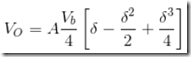" /> Compensation Techniques Thermistor | TN Mikro
Home > English > Compensation Techniques Thermistor

# Compensation Techniques Thermistor

Characteristics thermistor below shows the relationship between temperature and resistance as shown in the following figure. To control the need to change prisoners into stress, the following basic circuit to turn resistance into voltage.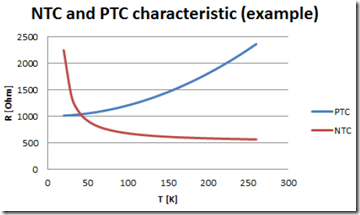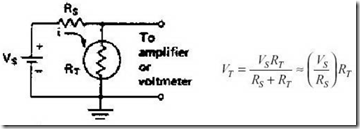Thermistor with a positive coefficient (PTC, Positive Thermal Coeffisien) Graph characteristics PTC thermistor type: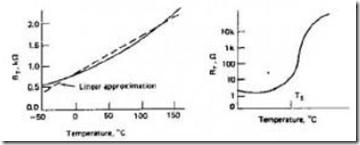In operation utilizing PTC thermistor type resistivity change with temperature, and generally the value prisoner rises exponentially with temperature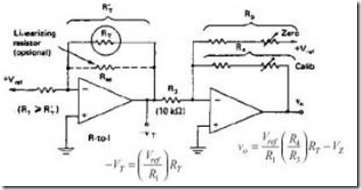Linear resistance region approaching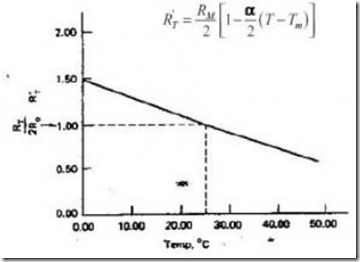For temperature compensation technique using a bridge amplifier circuit is better used for this type of sensor resistance as a bridge circuit can set its equilibrium point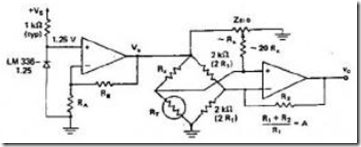The value of the output voltage is: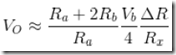Or other formulas that can be used to determine the output voltage: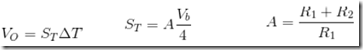thus: